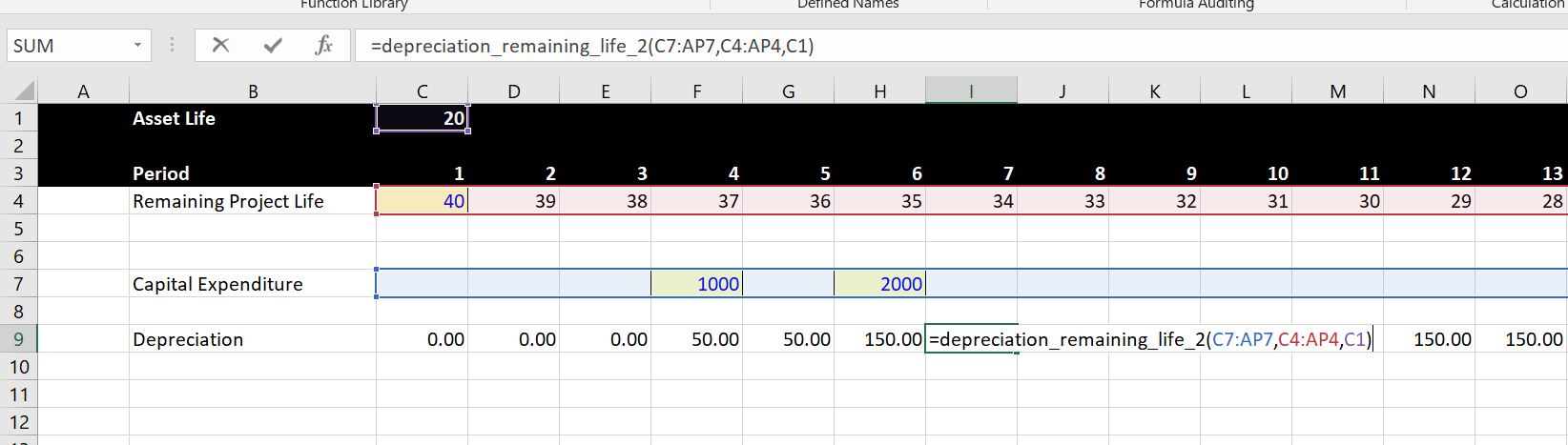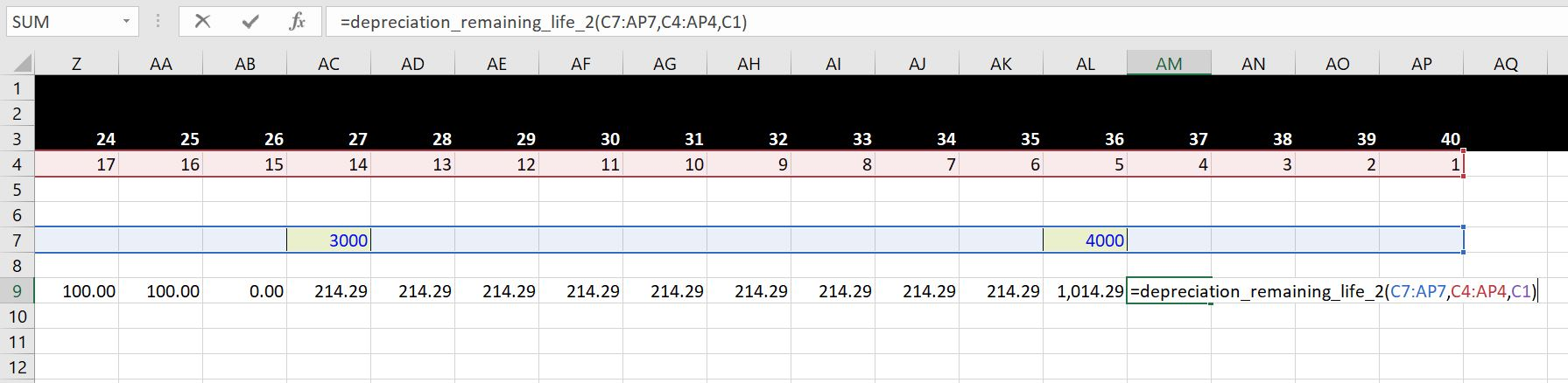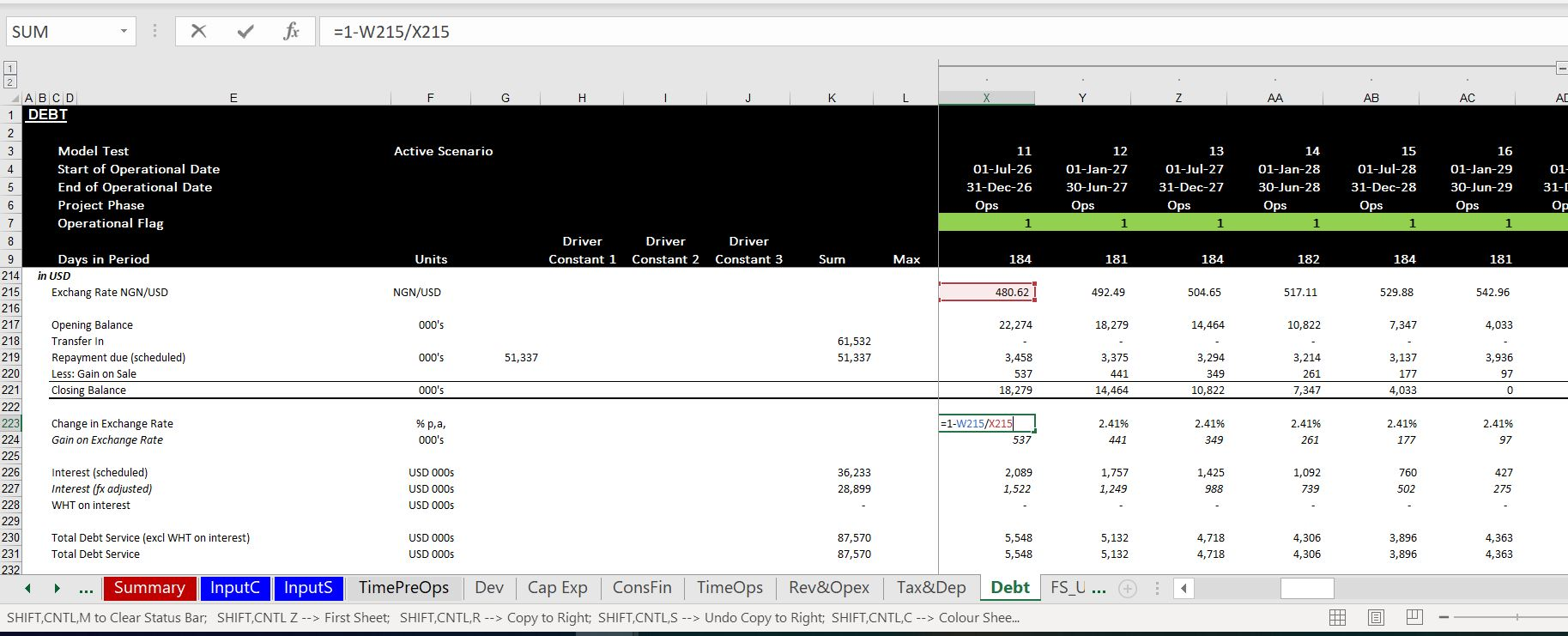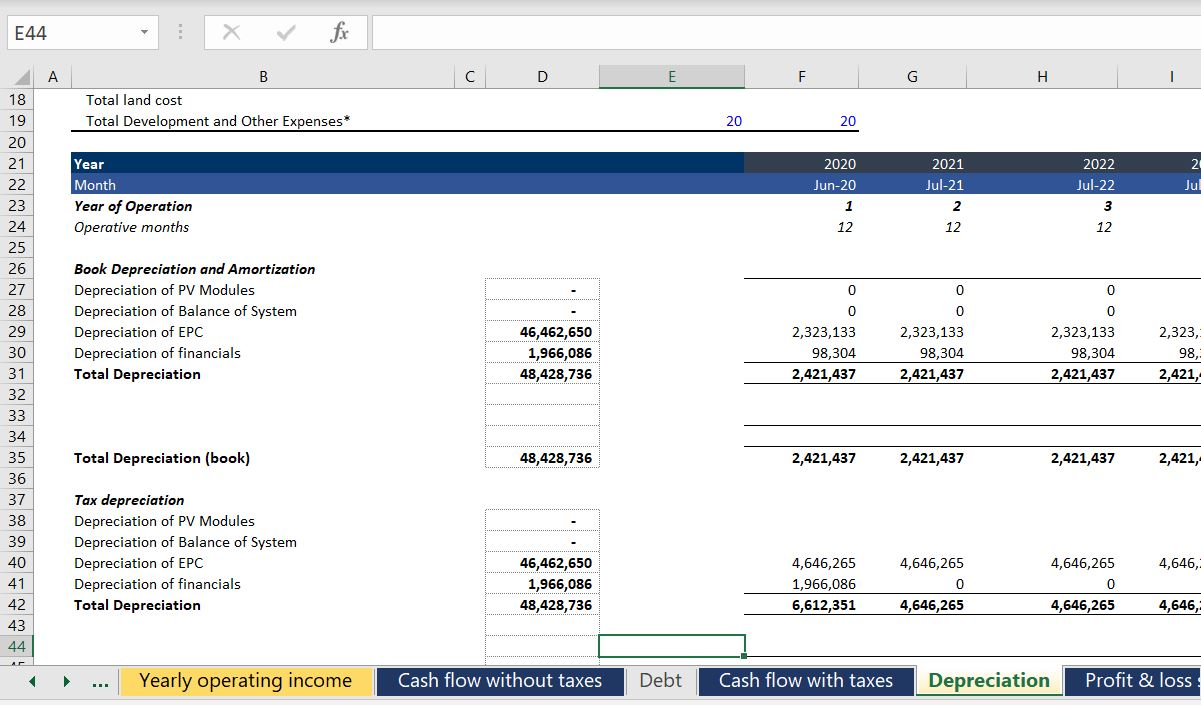# Operating, Tax and Depreciation

In a model, whether an agriculture model, a model of a toilet paper factory in Jamica, a model of an airport or an electricity project, do not start with any thing that has money (Euro, USD, CFA etc.) Instead, start with the capacity and volumes and what the machine or the project is really doing.  This is the subject of the second part of the course. In this section the INTERPOLATE UDF function is introduced to illustrate how to make volumes, capacity factor or other items gradually increase or decrease (a simple way to interpolate is not in excel).  You can download a file with the interpolate function below so that you can add the function to any of your models and do interpolate really fast.

Problems with not using lookup or interpolate_lookup are illustrated in the screenshot below.  In this case somebody thought they were really cool by using MATCH and INDEX together.  But this just creates a long mess.  It is much better to use the LOOKUP function with an entire line.## Pre-Tax Operating Cash Flow

The third part of the course begins with some equations that involve money.  In any project from a PPP for a University, a toll road, a co-generation plant or any electricity project, you should start with EBITDA and Capital Expenditures (and, maybe working capital).  These items define the pre-tax project IRR of the project.  With the pre-tax project IRR you have the essential information on the project.  In this page I use the IRR even though I believe there are a number of problems with the IRR.  To see a discussion of problems with the IRR you can go to my separate page that deals with the subject.

## Depreciation and Taxes to Compute Project IRR After Tax

The second set of videos complete the model with no debt and begin adding debt to the models. The last video in this section begins to add a circular resolution template to the model. This is an important idea in project finance where you would like to maintain flexibility in the face of natural circular references.

In adding on-going depreciation on future capital expenditures, I have included a function that enables you to select the entire line of future capital expenditures and remaining lives.  With these data items and the lifetime of the equipment, you can derive the depreciation on future capital expenditures.  The future depreciation is either from standard depreciation if the lifetime is greater than the remaining life.  Alternatively it is computed from the remaining life when the remaining life is less than the asset life.  A file that contains the function is available for download by pressing the button below (note that you have to press Shift, CNTL, Enter instead of enter and you cannot use the entire line).  The file also contains a function that is more flexible and will simulate variable declining balance depreciation.

The screenshots below illustrate how to use the function with straight line depreciation.  The first screen shot demonstrates how to function works in the case where the remaining life is greater than the asset life and the asset can be fully depreciated over the remaining life. In this case, the capital expenditure occurs in year 20 and the remaining life is 34 years. Note that two capital expenditures are entered and the depreciation stabalises at a level of 150.  After the first asset is retired in period 24, the depreciation will fall to 100. The second screen shot shows the case where the remaining life is less than the asset life. In this case the depreciation is computed over the remaining life of the asset.  The inputs for this function include the remaining life, the capital expenditure array and the asset life as shown in both screen shots.The video below works through various depreciation and issues related to future capital expenditures and explains why the problem of future capital expenditures and depreciation would be very messy without a function.  You would have to make one of those diagonal matrices and adjust for situations where the depreciation would expend beyond the life of the project.

.

.

The VBA code for the depreciation function is available for download below. Because this is a function, you must copy the code into your model rather than simply having the file with the VBA code open (with regular old macros you can do this). You can get this function with the VBA code into your project finance model in three different ways:

1. The first way is to open the file above and press ALT, F8.  Then edit the VBA page with the subroutine named A_Depreciation_functions.  Copy the entire contents.  After that, go to your sheet and press ALT, F8 again.  Then create a blank VBA subroutine and copy the contents above that routine.
2. The second way is to copy the function that is on the form that appears when you open the file.  You just copy the code, go to your sheet, press ALT, F8, create a dummy macro and paste.
3. The third method is similar to above, but you just copy the code from below.
```Option Base 1

'''''''''''''''''''''''''''''''''''''''''''''''''''''''''''''''''''''''''''''''''''''''''''''''''''''''''''''''''''
'''''''''''''''''''''''''''''''''''''''''''''''''''''''''''''''''''''''''''''''''''''''''''''''''''''''''''''''''''
'''''''''''''''''''''''' Depreciation Functions
'''''''''''''''''''''''''''''''''''''''''''''''''''''''''''''''''''''''''''''''''''''''''''''''''''''''''''''''''''
'''''''''''''''''''''''''''''''''''''''''''''''''''''''''''''''''''''''''''''''''''''''''''''''''''''''''''''''''''

Sub A_Depreciation_Functions()

End Sub
Function depreciation_remaining_life_2(capital_expenditure, remaining_life, max_life) As Variant ' When the output is an array define as Variant

cap_exp_periods = capital_expenditure.Count ' See how many capital expenditure periods are modelled

Dim Depreciation_Expense(5000) As Single ' Make a new array variable that is the output
Dim remaining_life1(5000) As Single

' Determine whether to use remaining life or something shorter

For vintage = 1 To cap_exp_periods ' make a second loop to evaluate asset by asset
If remaining_life(vintage) >= max_life Then
remaining_life1(vintage) = max_life
Else
remaining_life1(vintage) = remaining_life(vintage)
End If

Next vintage

For model_year = 1 To cap_exp_periods ' loop around each period and make a square with columns and rows

For vintage = 1 To cap_exp_periods ' make a second loop to evaluate asset by asset

age = model_year - vintage + 1 ' calculate the age of each expenditure (the diagonal)

If (age > 0 And remaining_life1(vintage) <> 0 And remaining_life1(vintage) >= age) Then ' Only when asset is alive

Depreciation_Expense(model_year) = _
capital_expenditure(vintage) / remaining_life1(vintage) + Depreciation_Expense(model_year)
End If

Next vintage ' Note that the vintage is used for the capital expenditure

Next model_year

depreciation_remaining_life_2 = Depreciation_Expense

End Function

.```

## Balance Sheet and Verification before Financing

The balance sheet is a good (but not perfect) way to check your model.  A few years ago a student in my class told me that he puts a balance sheet into the model very early on in the process. At first I did not see the value in this. With complex models and solving circular references I think that suggestion was exactly on the mark.  So much so that I put a test right at the top of the model and believe the saying on the t-shirt below from my friend Hedieh (you can order this t-shirt or a mug if you want. Just send an e-mail to edwardbodmer@gmail.com).You can put the balance sheet into the model after you have modelled the depreciation expense.  The balance sheet should just collect closing balances from other sections of the model. The video below describes the process of balancing the balance sheet before any debt had been put into the model.

This page includes an exercise where you build the financing part of a project finance model (i.e. starting with EBITDA) and then moves to the issue of resolving circular references. The first part of this page provides instructions on how to build the financing part of a model with flexible construction financing (pro-rata or equity first), sculpting of debt and a funded DSRA account.  The exercise includes an associated video that explains how to work through debt sizing, repayment, interest and the DSRA from a file that includes blank titles. I use this exercise as a pre-course assignment in project courses that are advanced where I deal with nuanced issues of debt sizing, debt funding, debt repayment, debt cost of capital and debt protections.  In these courses I don’t want to take time building an A-Z model and participants can assure themselves that they have the fundamental modelling skills. As this exercise includes circular references for IDC, DSRA and sculpting with taxes, a demonstration of how to implement the parallel model concept and resolve circular references is included. In order to focus on the tricky project finance issues, the exercise is for a case with a single debt issue in the context of an annual model. For the pre-course eercise, you should only focus on the first part of this page.

## Depreciation and Different Currencies

Many times the financing and pricing of your project will be in Euro or USD, but the project is located in a country and pays taxes based on the local currency. In these cases the tax should reflect the exchange rate and you need to make translation adjustments to get the balance sheet correct.  When making exchange rate adjustments, instead of using a percent change with this period currency/last period currency – 1, you go the other way.  If you use the formula 1 – prior period/this period as shown in the screenshot below and also use the opening balance of a debt account or an asset account, the exchange rate adjustments will make the balance come to zero at the end of an asset life.  This is illustrated below.## Who Cares about Book Depreciation in Project Finance

I notice that the depreciation is overly complex in many models.  One problem is why even bother with book depreciation in project finance models.